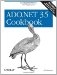# Using Expression Columns to Display Calculated Values

Problem

You need to display a calculated value for each row in a DataTable and to filter and sort the table on this value.

Solution

Add an expression column to the table and display it. Sort and filter on the expression column from the default DataView for the table.

The sample code contains two event handlers:

Sets up the sample by creating a DataTable containing the Order Details table from the Northwind sample database. An expression column calculating the extended price for each detail line is created and added to the table. Finally, the default view for the table is bound to the data grid on the form.

Apply Button.Click

Applies the filter and sort order entered by the user to the Extended Price expression column in the data view.

The C# code is shown in Example 3-2.

Example 3-2. File: ExpressionColumnForm.cs

```// Namespaces, variables, and constants
using System;
using System.Configuration;
using System.Windows.Forms;
using System.Data;
using System.Data.SqlClient;

// . . .

private void ExpressionColumnForm_Load(object sender, System.EventArgs e)
{
// Define the table and fill it with data.
DataTable dt = new DataTable("OrderDetails");
String selectText = "SELECT * FROM [Order Details]";
ConfigurationSettings.AppSettings["Sql_ConnectString"]);
da.Fill(dt);

// Add an expression column to the table.
"(Quantity * UnitPrice) * (1 - Discount)"));
// Define the DataView object.
dv = dt.DefaultView;

// Bind the DataView to the DataGrid.
resultDataGrid.DataSource = dv;
}

private void applyButton_Click(object sender, System.EventArgs e)
{
bool isLowerBound = false;
bool isUpperBound = false;

Decimal lowerBound = 0;
Decimal upperBound = 0;

if(filterLowerBound.Text.Trim( ).Length > 0)
{
isLowerBound = true;
try
{
lowerBound = Decimal.Parse(filterLowerBound.Text);
}
catch(System.FormatException)
{
MessageBox.Show("Invalid entry for lower bound.",
"Error", MessageBoxButtons.OK,
MessageBoxIcon.Error);
return;
}
}

if(filterUpperBound.Text.Trim( ).Length>0)
{
isUpperBound = true;
try
{
upperBound = Decimal.Parse(filterUpperBound.Text);
}
catch(System.FormatException)
{
MessageBox.Show("Invalid entry for upper bound.",
"Error", MessageBoxButtons.OK,
MessageBoxIcon.Error);
return;
}
}

String filter = "";
if(isLowerBound)
filter = "ExtendedPrice >= " + lowerBound;

if(isUpperBound)
filter += ((isLowerBound)?" AND " : "") +
"ExtendedPrice <= " + upperBound;

// Set the filter.
dv.RowFilter=filter;

// Set the sort.
dv.Sort = "";
dv.Sort = "ExtendedPrice";
dv.Sort = "ExtendedPrice DESC";
}
```

Discussion

An expression column contains a value that is calculated from other column values in the same row, or from an aggregate of rows in the table or in a related table. The DataType of the column must be compatible with the return value of the expression. For information about expression syntax, see the "DataColumn.Expression Property" topic in the MSDN Library.

An expression column is added to a table either through one of the DataColumn constructors that take the expression for the column as the third argument (the technique used in the sample code) or by setting the Expression property of the column to the expression.

In the sample, an expression column named ExtendedPrice is created with a data type of Decimal . The column calculates the extended price for the column using the expression (Quantity * UnitPrice) * (1 - Discount) .

After the column is added to the table, the RowFilter and Sort properties of a DataView bound to the table with the expression column can sort or filter data in the same way as they can on any other column. This is shown in the sample code and discussed in more detail in Recipe 3.1.